Select Page

# Depreciation

Previous Lesson: Final Accounts (Non-Corporate)

Next Lesson: Joint Stock Company

Plant assets (also called property, plant, and equipment) are resources that have physical substance (a definite size and shape), are used in the operations of a business, and are not intended for sale to customers.

The cost principle requires that companies record plant assets at cost. Cost consists of all expenditures necessary to acquire an asset and make it ready for its intended use. If a cost is not included in a plant asset account, then it must be expense immediately. Such costs are referred to as revenue expenses. Costs that are not expense immediately, but are instead included in a plant asset account are referred to as capital expenditures. Once cost is established, it becomes the basis of accounting for the plant asset over its useful life.

Depreciation is a non-cash expense. The term depreciation is used with reference to tangible fixed assets because the permanent continuing and gradual fall in book value is possible only in the case of fixed asset. The value of assets gradually reduces on account of us Such reduction in value is known as depreciation.

• Expired cost of an asset is called depreciation
• Depreciation is the process of allocating to expense the cost of a plant asset over its useful (service) life in a rational and systemic manner
• Such cost allocation is designed to properly match expenses with revenues.
• Depreciation affects the balance sheet through accumulated depreciation, which is reported as a deduction from plant assets
• It effects the income statement through depreciation expense

Depreciation is a cost allocation, process not an asset valuation process. The book value—cost less accumulated depreciation—of a plant asset may differ significantly from its market value. Land is not a depreciable asset. Recognizing depreciation for an asset does not result in the accumulation of cash for replacement of the asset. The balance in the accumulated depreciation account represents the total amount of the asset’s cost that the company has charged to expense to date. Factors in computing depreciation:

• Cost—Plant assets are recorded at cost, in accordance with the cost principle
• Useful life—an estimate of the expected productive life, also called service life, of the asset
• Salvage value—an estimate of the asset’s value at the end of its useful life

## 1. Depreciation Methods

There are many methods used for calculating depreciation. All these methods help to ascertain an estimated amount of depreciation to be charged to the revenue of the year. Once a method is chosen, it should be applied consistently over the useful life of the asset. Some of these method are discussed below:

### 1.1 Straight Line Depreciation

Allocates an equal amount to each year of the asset’s expected life. The basic idea is that straight-line recognized that same amount of depreciation per year. Whatever that year. Under the straight-line method, an equal amount of depreciation expense is recorded each year of the asset.

#### Straight Line Depreciation Formula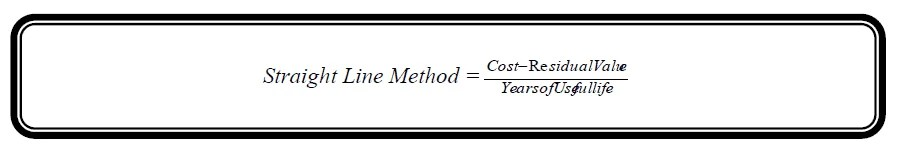### Example # 1:

On 1st, January 2005, Machine worth Rs. 20,000 was purchased. The estimated useful life of this is 4 years and scrap value is estimated Rs. 2,000.

Find the amount of annual depreciation under straight Line Method and prepare Schedule of Depreciation?

Solution: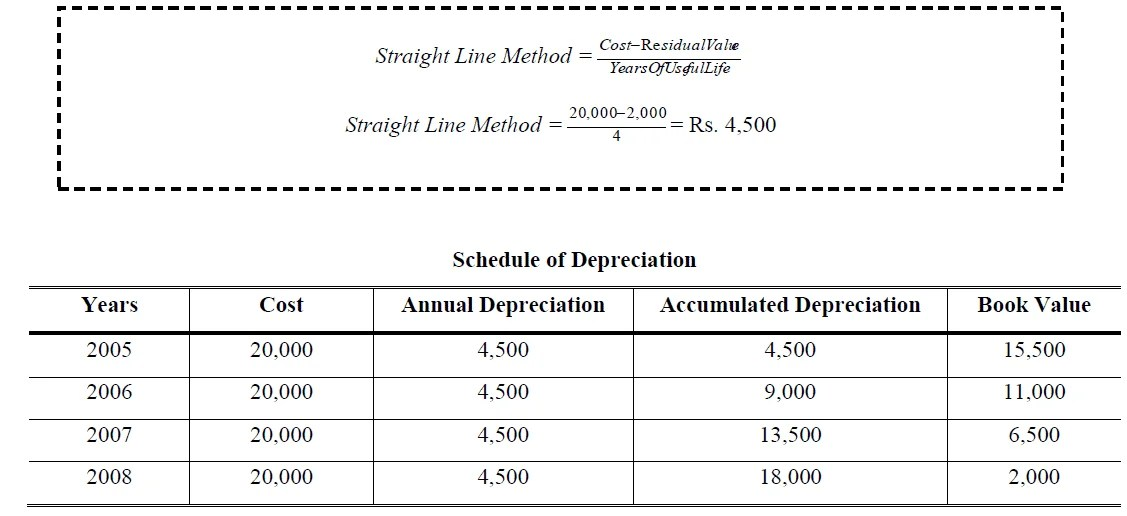### 1.2. Units of Production Depreciation

Under this method depreciation is calculated on the basis of use or productivity of the asset. If asset is used more or gave more productivity in  any year more depreciation charged and vice versa. Under the units-of-activity method, the life of an asset is expressed in terms of the total units of production or the use expected from the asset. The units-of-activity method is suited to factory machinery and such items as delivery equipment and airplanes. This method is generally not suitable for such assets as buildings or furniture because activity levels are difficult to measure:

#### Units of Production Depreciation Formula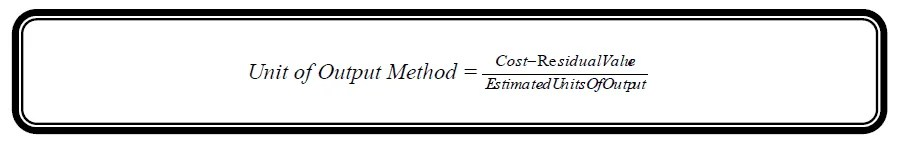### Example # 2:

On 1st, January 2005, a delivery truck was purchased for Rs. 400,000. The estimated working life is 200,000 kilometers and salvage value is Rs. 100,000. The truck covered 40,000 in 2005, 50,000 kilometers in 2006, 35,000 kilometers in 2007, 32,000 kilometers in 2008 and 43,000 kilometer in 2009. Calculate the rate of depreciation per kilometer and prepare Schedule of Depreciation

Solution: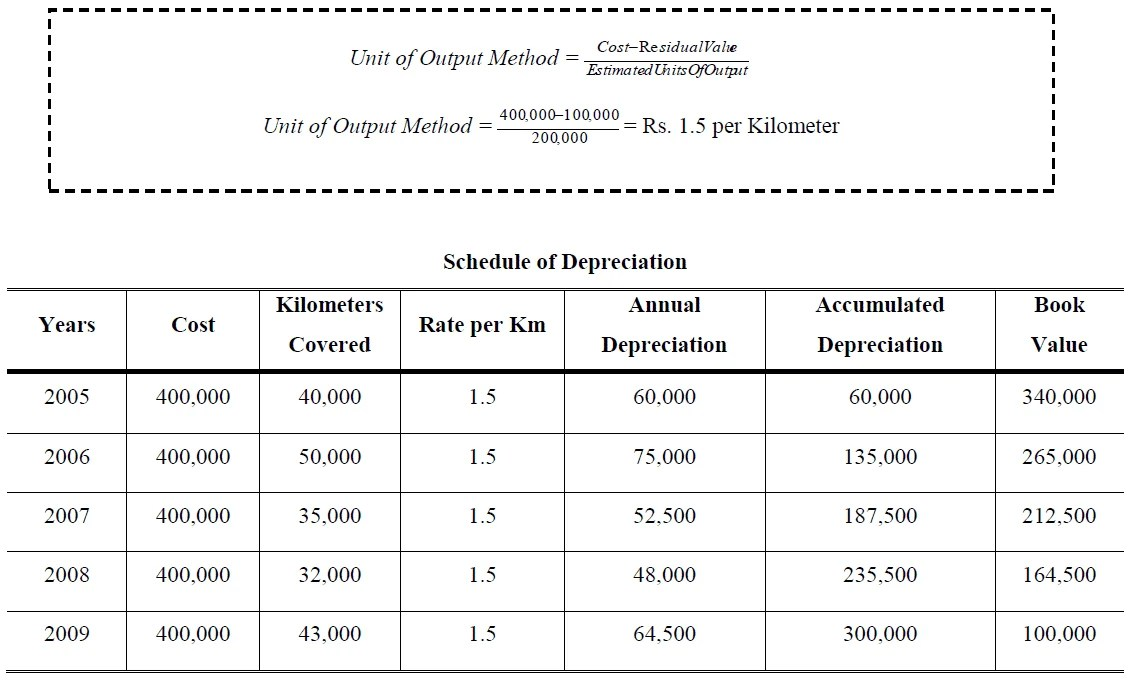### 1.3. Declining Balance Method

Under this method the asset is depreciated at fixed percentage calculated on the book value of the asset which is diminished year after year on account of depreciation. This method associates the high amount of depreciation expenses in the initial years and low amount in the later years. The yearly rate of depreciation is calculated as under:

### Example # 3:

On 1st, January 2005, Machine worth Rs. 1,000,000 was purchased. The estimated useful life of this is 3 years and residual value is estimated Rs. 64,000. Find the rate of depreciation under Declining Method and prepare Schedule of Depreciation

Solution:### 1.4. Double Declining Depreciation

This method is modification of decline balance method to make it simple. The calculating rate of depreciation is discussed below:

• Calculating the depreciation rate (1/ Expected Life)
• The rate calculating above is multiply by 2. This is called double decline rate
• Last year’s depreciation is calculated by deducting the residual value from book value

### Example # 4:

On 1st, January 2005, Machine worth Rs. 40,000 was purchased. The estimated useful life of this is 5 years and residual value is estimated Rs. 3,000. Calculate the depreciation expense of machinery by Double Declining Balance Method and prepare Schedule of Depreciation.

Solution:### 1.5. Sum of the Years Digits

Sum of the Years’ Digits Method is also based on the assumption that the loss in the value of the fixed asset will be greater during the earlier years and will go on decreasing gradually with the decrease in the life of such asset. The SYD is found by estimating an asset’s useful life in years, then assessing consecutive numbers to each year, and totaling these numbers i.e. SYD = 1 + 2 + 3 + 4 + …… +. Depreciation is calculated on cost minus residual value (Written off value).

### Example # 5:

ABC Ltd. purchased a truck for Rs. 65,000 on 1st January 2001. The expected life was 5 years and salvage value Rs. 5,000. Calculate the annual depreciation expense by applying sum-of-the-years’ digits (SYD) method.

Solution: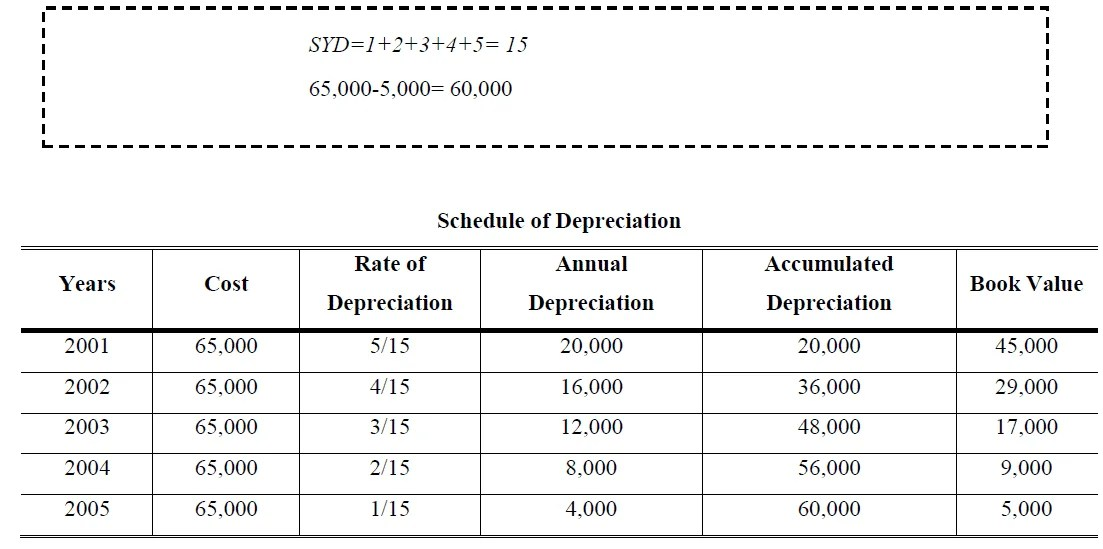### 1.6. Modified Accelerated Cost Recovery System (MACRS)

The MACRS allows a firm to write off a big portion of an asset’s book value early in the life of the asset. The IFRS has classified assets into different classes according to their expected life spans. The following table shows the different classes of assets (according to an asset’s life span)### Example 6:

Orange Computer Inc. bought a new computer for Rs. 25,000 in 1995. Orange Computer paid an additional Rs. 2,500 for shipping and installation costs. What is the depreciation value of the computer over the life of the computer?

Solution: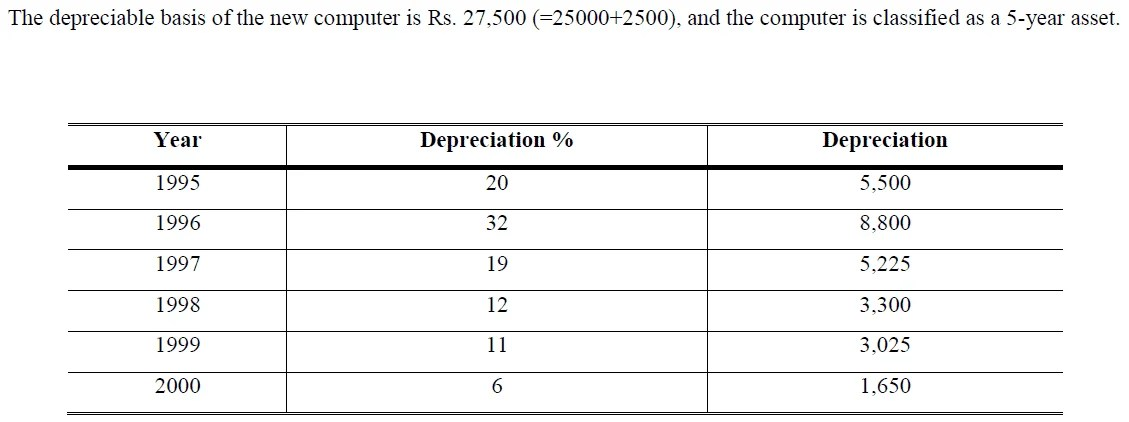## References

Mukharji, A., & Hanif, M. (2003). Financial Accounting (Vol. 1). New Delhi: Tata McGraw-Hill Publishing Co.

Narayanswami, R. (2008). Financial Accounting: A Managerial Perspective. (3rd, Ed.) New Delhi: Prentice Hall of India.

Ramchandran, N., & Kakani, R. K. (2007). Financial Accounting for Management. (2nd, Ed.) New Delhi: Tata McGraw Hill.

1. Very interesting…

2. good work

3. Saved as a favorite, I love your web site!

4. My sister bookmarked this internet site for me and I have been going through it for the past several hrs. This is really going to help me and my classmates for our class project. By the way, I like the way you write.

5. Pls attach z full doc